The Universe: Events and Waves

To any observer, the universe is one gigantic on-going event, comprising a continuous stream of consciously demarcatable features, borne as indelible etchings within the fabric of time, converging from every direction, into the sink-holes at the centres of the atoms of his eyes. [Português]

The consciously demarcatable features, of the great event we call the universe, are what we see as the separate events taking place within it. Each such event etches its mark upon the fabric of time, which I postulate to be a universal essence called the æther. The æther flows, like an infinity of unseen 3-dimensional conveyor belts, bringing sense of the event convergently towards each observer.

Science perceives what an event etches upon the æther as a wave. This is probably because, in a lot of ways, it seem to behave like a wave in water or air, which can be seen and felt directly. A wave in water can be seen in transit. Experiments [such as Kundt's Tube] can reveal a sound wave in transit through air. Such a wave can be indisputably observed to be divergent. It diverges, in every direction, away from the event that created it. Notwithstanding, I think that what I shall refer to as a wave in the æther is fundamentally different in that, instead of diverging away from its source, it converges towards its sink [the observer].Æther is continually converging towards, and dis­appearing into, every observer at velocity, c, as de­picted by the animation on the right. As an event occurs, it etches its mark upon the passing æther, in the way the swinging pen of a chart recorder writes its trace upon the moving paper. The appar­ent size of the event is determined by the solid angle it subtends from the observer's point of view. The intensity of the event is determined by the depth to which the event inscribes itself upon the æther. The intensity of the inscription is deter­min­ed by the extent to which the event polarises the æther in some as yet unspecified way.

Any event, other than the universe itself, has a finite lifespan. It has a beginning, a middle and an end. During its finite life, it is continually writing its trace upon the fabric of time, namely, the passing æther. Once its life is finished, it ceases to exist — at least, as an active agent. Yet its whole history is etched upon the æthereal inflow of each observer, to be unfolded before his eyes at some future date.

But how does an event write a record of its occurrence onto the æther? To answer this, it is first necessary to answer the pending question about where the energy goes that is expended by a directed external force in accelerating an object against its inertial reaction.

Force, Mass and Acceleration

To investigate this, we must look again at the notions of force, mass and acceler­ation in a somewhat different light. What is humanly perceived as acceler­ation [rate of change of velocity] embraces two distinct phenomena. These are:

1. relative acceleration, and
2. absolute acceleration.

Absolute acceleration requires an externally-applied directed force: relative acceler­ation does not.

The most straight-forward example of relative acceleration is when two objects pass each other on a non-collision course in simple 3-dimensional Euclidean space. Each object is moving at constant velocity along its own straight course. Each is there­fore at rest with respect to the æther. It is suffering no external applied force. Yet from the point of view of one of the objects [the observer], the other object acceler­ates towards him during its approach, passes by, and then decelerates as it recedes from him. Furthermore, the acceleration and deceleration concerned are extremely non-linear. Consequently, the fact that an object appears to be acceler­ating does not necessarily mean that it is being acted upon by a directed external force. In other words f = ma ain't necessarily so.

Absolute acceleration is caused when an object is momentarily perturbed, by a dir­ected external force, from its state of rest with respect to the æther. An object, in this context, comprises one or more æthereal sink holes and the polarized æther which surrounds them. In other words, an object is a solid com­posite (such as a ball bearing) which comprises one or more atoms or components of atoms.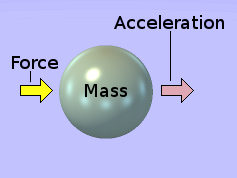Imagine a solid sphere in free space, as shown in green on the left. An external directed force is app­lied to the sphere, as indicated by the yellow arrow. This causes the sphere to accelerate as indicated by the pink arrow. The degree to which the sphere ac­celerates is directly proportional to the amount of force applied to it. The constant of pro­portionality that relates the rate at which the sphere acceler­ates, under an applied force of a given mag­nitude, is called the sphere's mass.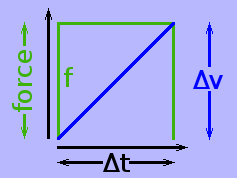Suppose the force is kept at a constant value and is applied for an amount of time δt. The force 'f' is shown graphically by the green square-wave on the right. It rises instantly to its full value, remains at that value for the prescribed amount of time, Δt, then drops back to zero instantly. The velocity, v, of the sphere increases at a constant rate, as shown by the blue line. During the small period Δt, throughout which the force is applied, the sphere's velocity in­cr­eases by Δv.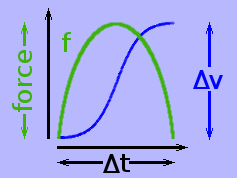In reality, nature does not readily facilitate the inst­antaneous rise of a force from zero to a tangible val­ue, the way I have shown it above. In the real world, the rise and fall of the force would generally be sin­usoidal, with the velocity of the object following a sigmoidal curve. After the sphere has undergone its short burst of acceleration, its relative velocities, with respect to all other objects in the universe, will have changed by an amount Δv.

Of course, the sphere's change in velocity, relative to any other specific object in the universe, is the resolved component of Δv [namely, Δv × cos(θ)] in the direction of that object, where θ is the angle between the path along which the sphere was accelerated and the bearing of the object from this path.

The actual value, v, of the sphere's relative velocity — whether before or after the acceleration — has little significance. For instance, if we were in a frame of reference in which the sphere's initial velocity were enormous in the left-ward direction, then the force shown would be a decelerating force, which would be perceived as merely slowing the sphere down a bit.

Consequently, the sphere's relationships with all other objects in the universe have changed. Its relationship with the rest of the universe has changed. Thus, a change has occurred. A force has been applied through a distance. A dissipative event has taken place. Energy has been exp­ended. But how much?

Energy Expended by The Force

To answer this question, it is necessary to begin with 2 definitions, which are really artificial conventions adopted by scientists. The first definition is that acceleration is the rate of change of velocity with time, i.e.:

acceleration = Δ(velocity) ÷ Δ(time).

The Δ prefix signifies a change as opposed to a measurement made with reference to an absolute datum. Thus Δ(velocity) signifies a change in the relative velocity of an object without reference to any designated "sta­tionary" object such as the Earth. Likewise, Δ(time) signifies a period of time (so many seconds) stated without reference to any time standard such as Greenwich Mean Time (GMT).

For the convenience of the following discourse, however, I would like to rearrange it as a definition of the time interval, Δ(time), over which a burst of constant acceler­ation takes place. The second is the definition of energy, or rather, a change in energy. The two definitions can therefore be written as:

1. Δ(time) = Δ(velocity) ÷ acceleration
2. Δenergy = force × Δdistance

To these I wish to add 3 fundamental scientific observations. Be well aware that these are direct observations of nature. They are not derived from anything else. This is important.

1. Δ(average-velocity) = Δ(velocity) ÷ 2
2. Δ(distance) = Δ(average-velocity) × Δ(time)
3. force = mass × acceleration

The variables prefixed with the Δ symbol are, by nature, relative quantities. They have no absolute zero. The variables without a Δ prefix are, by nature, absolute quantities. They have a zero value which is universally absolute. This distinction is very important. In this present context, each absolute variable will be considered to be held at a constant value.

By substituting expressions 3. and 1. respectively in expression 4., it is possible to derive, algebraically, a useful consequence, as follows.

 Δ(distance) = {Δ(velocity) ÷ 2} × Δ(velocity) ÷ acceleration 6. = ½{Δ(velocity)}² ÷ acceleration

By substituting expressions 5. and 6. respectively in expression 2., it is possible to derive an expression for the energy expended by a force when it is applied to an object for a duration Δ(time) through a space Δ(distance).

 Δ(energy) = mass × acceleration × ½{Δ(velocity)}² ÷ acceleration = mass × ½{Δ(velocity)}² 7. = ½mass × {Δ(velocity)}² [familiar expression for kinetic energy]

In my conception, mass is not an inherent property of the object being accelerated. It is simply a constant of proportionality relating Δ(energy) to Δ(velocity).

The above formula expresses the energy, Δ(energy), expended by the force, in terms of the change in velocity, Δ(velocity), of the sphere. To be able to calculate the energy, therefore, it would be necessary to be able to measure the sphere's change in velocity.

Within the context of the sphere, this would necessitate the presence of other obj­ects somewhere within the field of view for use as points of reference against which to gauge the sphere's change in velocity. Alternatively, the page could be con­sidered an observational frame of reference with the sphere itself moving within the field of view of the illustration.

Unfortunately, either of these options would complicate the situation enormously. A more fundamental approach is required.

A Minimalist Approach

To construct this more fundamental view, it is necessary to make a simple state­ment, which is true, although counter-intuitive within the context of human experi­ence in a terrestrial environment:

Velocity is not a property of an object. It is a property of the relationship between two objects.

This is why the adjective relative so often prefixes the word velocity. The same is true of distance, which is merely the special case where the relative velocity be­tween two objects is zero.

For instance, to say that the Earth has a certain velocity of so many thousands of kilometres per hour is meaningless without some qualifying addendum. Over any short time interval Δt, the Earth has one velocity relative to Venus, a different velo­city relative to Mars, another velocity relative to Jupiter, and so on for every other object in the universe. Consequently, from Venus' point of view, the Earth has a certain amount of kinetic energy. From Mars' point of view, the Earth has a different amount of kinetic energy. From Jupiter's point of view, the Earth has yet another amount of kinetic energy, and so on for every object in the universe.

As I said, the above considers the case over a very short time interval Δt. In the long term, as the Earth and Mars move round their orbits, the relative velocity of their approach or recession is substantially non-linear. Hence, in the long term, the Earth's kinetic energy, from the point of view of Mars, varies non-linearly. And it follows, therefore, that so does its kinetic energies relative to all the other planets.

Such non-linear changes in relative kinetic energy cannot be accounted for through any notion of changes in what scientists term potential ener­gy. This is evinced by the passive acceleration, which takes place when two bodies approach then recede from each other when passing on a non-collision course in free space. Here, in the absence of gravity or orb­its, the relative velocity — and hence the relative kinetic energy — be­tween the two bodies varies non-linearly without any interaction taking place between the two bodies. No potential energy is involved.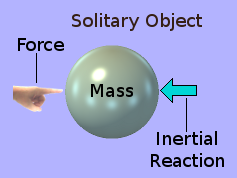In order to get to the heart of the matter of an ob­ject's relationship with the universe, it is neces­sary to consider a solitary object in space, with no other object within sight or influence. In other words, the object cannot be examined from the point of view of any frame of reference other than its own. The ob­ject itself is its own observer. Let us suppose that a force is applied by the Finger of God, to which the object reacts with an equal and opposite inertial re­action.

I use the Finger of God to signify a force which is supplied from outside the context of the physical — i.e. the measurable — universe. This force is applied to the ob­ject, namely, the green sphere. The object — as the observer — experiences the force at one point on its left side. From this point, the rigid structure of the object transmits the force to all its parts. The force is thus distributed from a point on the object's surface to all its parts. On the other hand, the inertial reaction to the force emanates from every part of the object. It is transmitted from each part of the object, through the object's rigid structure, to the single point on its left side at which God applied his Finger.

I have not included in the above illustration any indication that the object, as a result of the applied force, is in a state of acceleration. This is because, in order to acquire a notion of acceleration, it is necessary to refer to other objects. And in this present context, there are no other objects within sight or influence. The object is alone in the universe. The universe is infinite. The universe is made of æther. The object is immersed in an infinite sea of æther.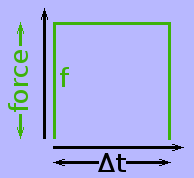Within this present context, therefore, the object — who is also its own observer — can have no notion of velo­city, space or even acceleration. So what is left? How can the observer be consciously aware of anything? The answer is time. And time is a very difficult notion to get a handle on. However, if the observer has any conscious sense at all, he can experience the event of the force being app­lied to the object in the first place and also the event of its cessation, i.e. when the force is withdrawn or removed from the object.

In this minimalist situation, the observer can experience the square-wave event illustrated above on the left. However, this necessitates that the observer have a sense of time. He must be able to experience ­ and hopefully measure — the pas­sage of the period Δt and be aware that there was an epoch pre-dating the applic­ation of the force and an epoch post-dating its cessation. As with distance, velocity and acceleration, time can only be measured with respect to a recognizable object of reference. In other words, the observer needs some kind of reference clock that emits regular tick signals. In the terrestrial environment, day and night, moon phases and the annual seasons provide natural clocks. However, in this mini­malist situation, the observer would need to use his heartbeat or some kind of arti­ficial clock in order to gauge the duration of Δt.

In this minimalist context, of a solitary object in space with no other object within sight or influence, it is possible to construct a temporal frame of reference. It is even possible, thereby, to construct a means of measuring the rate of passage of time. To do this, we must divide the sphere into two separate concentric spheres. We set the small inner concentric sphere rotating. We keep the outer concentric spherical shell rotationally stationary. The rate of rotation of each can be gauged by the magnitude of the static centrifugal force within its material. For simplicity, let us assume that the Fingers of God exert a torque upon the inner sphere for a given period of time in order to set it spinning. Each time a mark on the outer surface of the rotating sphere passes a corresponding mark on the inner surface of the spher­ical shell defines one standard time period. Thus we can measure Δt.

This Energy In Terms of Time

Unfortunately, in this minimalist context, the observer has no sense of — or means of measuring — the increase in relative velocity, Δv, of the object. Consequently, the observer cannot determine the energy expended (work done) by the force ex­erted by the Finger of God. However, since he can measure the duration Δt of the force's action, he can determine the object's change in momentum, Δ(momentum), viz:

9. Δ(momentum) = force × Δ(time)

But momentum can also be expressed as:

Δ(momentum) Δ(velocity) = mass × Δ(velocity) 10. = Δ(momentum) ÷ mass

The energy, Δ(energy), expended on the object by the force is

 Δ(energy) = ½(mass) × {Δ(velocity)}² from equation (7.) = ½(mass) × {Δ(momentum) ÷ mass}² substitute equation (10.) = ½{Δ(momentum)}² ÷ mass. = ½{force × Δ(time)}² ÷ mass substitute equation (9.) 11. = ½{force² ÷ mass} × {Δ(time)}²

I like to conceptualize mass as a coefficient of inertia per sink-hole, k, times the number of sink-holes, n, within the object. Thus m = k × n. The amount of force applied to the whole object is n times the force, δ(force), applied to each of the n sink-holes within the object. So δ(force) = force ÷ n. Equation (11.) above thus be­comes:

 Δ(energy) = ½{[δ(force) × n]² ÷ (k × n)} × {Δ(time)}² = ½{δ(force)}² × n² ÷ k ÷ n × {Δ(time)}² = ½{n² ÷ k ÷ n} × {δ(force)}² × {Δ(time)}² = ½{n ÷ k} × {δ(force) × Δ(time)}² 12. = {n/2k} × {δ(momentum)}²

The energy, δ(energy), expended by the divided force, δ(force), upon each æther­eal sink-hole for the same duration of time, Δ(time), is one nth part of the energy ex­pended by the full force upon the whole object:

 δ(energy) = {1/2k} × {δ(force)}² × {Δ(time)}² = {1/2k} × {δ(force) × Δ(time)}² = {1/2k} × {δ(momentum)}² 13. = ℵ × {δ(momentum)}²

Equation (13.) states that the energy, δ(energy), expended by the force, over the period Δ(time), upon each sink-hole within the object is proportional to the square of the resulting change in momentum, δ(momentum), of each sink-hole during that period, Δ(time). The constant of proportionality, ℵ = 1/2k, is a universal constant relating to the nature of the æther.

From the change in the sink-hole's momentum, δ(momentum), it is possible to ex­press the energy, δ(energy), imparted by the force δ(force), to the sink-hole in terms of the sink-hole's change in velocity, Δ(velocity).

 δ(energy) Since = {1/2k} × {δ(momentum)}² δ(momentum) = k × Δ(velocity) = {1/2k} × {k × Δ(velocity)}² = ½ × {1/k} × k² × {Δ(velocity)}² = ½ × k × {Δ(velocity)}² 14. = ℶ × {Δ(velocity)}²

I use the Hebrew letter ℶ to encapsulate distinctively the part of the equation that is simply a restructuring of the universal constant ℵ which, in turn, is simply a restructuring of what I have conceptualized as the coefficient of inertia of a single æthereal sink-hole. In other words, ℶ is a universal constant relating to the nature of the æther. Thus, by a simple re-rationalization of the units of measure, ℶ could be deemed dimensionless, giving energy the nature of a squared velocity.

This change in energy has the form of what mainstream science describes as the change in the object's inherent kinetic energy resulting from its increase in velocity. Notwithstanding, force, like temperature, is an absolute measure. It must always be measured from the same absolute zero. Consequently, energy expended by a force act­ing over a distance must also be an absolute measure. Velocity, on the other hand, is a relative measure. It can only be stated with respect to a specific observer or frame of reference. Consequently, kinetic energy also can only be stated with respect to a specific observer or frame of reference.

Absolute energy (force × distance) and kinetic energy (½mass × velocity²) are therefore measures of different stuff. The one cannot be converted into the other. Hence the energy expended by the force acting over a distance cannot become an increase in the object's kinetic energy.

Notion of Squared Velocity

I have difficulty in conceptualising square-velocity. In conventional LMT analysis a square-velocity would be L² T−2. It would be measured in square metres per square second. Square metres I can visualise. But square seconds are beyond me. There are obviously other ways of interpreting square-velocity. However, I prefer to think of it in the only way that makes sense to my spatial intuition.

Time is nearly always represented graphically by a length. It is generally shown as the horizontal axis on a graph. It is not surprising, therefore, that it eventually be­came thought of as a fourth dimension, which could be treated in exactly the same way as the 3 space dimensions. Notwithstanding, time is not conceptually the same as a space dimension. In fact, it has jurisdiction in all 3 dimensions of space.

Consequently, my intuition does not see time like a fourth dimension of space. The expression L² × T−2 is the same as L² ÷ T². It is a spatial area (a tangible notion) divided by a temporal area (an area of time), which I can't imagine. So, although the mathematical expression T² symbolizes a square in time, the tangible reality behind the symbolism must be radically different.

The expression L² ÷ T² is the same, mathematically, as L² ÷ T ÷ T, which would be measured in square-metres per second per second. This I can picture. I see an ex­panding area whose rate of expansion is increasing with time. In other words, the rate of growth in the size of the area is increasing at a constant rate.

If the object being accelerated is at a distance 'r' from the observer, the area of the sphere of radius 'r' centred at the observer, A = 4πr². Since the æther upon which the inscription is written, is falling towards the observer at velocity c, the area of the sphere de­creases as follows.

Area of the sphere A Rate of change of A = 4πr² = 8πr × dr/dt = c [which is a constant] = 8πr × c = 8πcr d²A/dt² = 8πc × dr/dt = 8πc × c = 8πc² [which is a constant]

The area of the sphere thus diminishes at a rate of 8πc² square metres per second per second. The spherical cap, bearing the æthereal inscription, is geometrically part of this sphere. Its area therefore collapses at the same rate of 8πc² square metres per second per second, as it falls towards the observer at velocity, c.

From this, I am able to acquire a conception of what square seconds are. They are, in this case at least, rates at which length is decreasing in two independent space dimensions, namely, decrements in Δ(latitude) and Δ(longitude) upon the spherical surface of the collapsing wave-front.

Side Note: In general, I don't see that the rates have to be the same in both the space dimensions concerned. They could be different in each. This invokes within my mind the notion that time can have as many in­dependent dimensions as can space, namely 3.

The constant velocity 'c', the velocity of light, is, according to currently accepted measurements, 299,792,458 metres per second. The area of the spherical wave-front must therefore collapse at the decelerating rate of 2·258818134×1018 square metres per second per second, as it speeds towards the observer. This certainly invokes within my mind the notion of the en­ergy of a wave falling 3-dimensionally, from its point of inscription into the æther, convergently towards the observer.

Kinetic Energy is Relative

Thus it would seem that what is perceived by conventional science as kinetic ener­gy has the geometrical form of a wave. So perhaps what is perceived as kinetic ener­gy is really æthereal wave-energy. If so, it is not imparted to the object by the force. The object merely has the passive role of transforming the linear energy exp­ended by the force into a wave within the æther. The object itself does not gain the imparted energy.

For example, a bullet fired from a rifle gives the impression that it inherently cont­ains kinetic energy gained from its propellant. Within a terrestrial environment, this is unquestionably intuitive. Notwithstanding, when considered in the context of the universe as a whole, the bullet has been decelerated by the propellant from the points of view of as many bodies in the universe as those from whose points of view it has been accelerated. Hence, its energy, relative to the universe as a whole has not changed.

The bullet's target, being in the terrestrial environment, happens always to be one of those objects in the universe from whose point of view it has been accelerated. The energy liberated on the bullet's impact with the target results from the intense momentary force applied, through a very small distance, to the bullet by the target. This energy is nothing to do with the energy expended by the force of the propel­lant in accelerating the bullet in the first place. It is a separate transaction.

Thus, although energy has been expended by the force in accelerating the green sphere, the sphere was at rest with respect to the æther before it was ac­celerated and it is again at rest with respect to the æther after it has been acceler­ated. Consequently, from the point of view of the sphere itself, its status quo is the same as it was before the event. So where is the effect of the event now? What has happened to the energy expended by the force?

During the period Δt, while the force is being applied, the object does not, as far as I am aware, suffer an increase in temperature. Consequently, any work being done by the force is not being accumulated by the object itself. So the inertial reaction cannot be resistive. It must be purely reactive. [I use these terms in the sense in which they are used in connection with electrical circuits.]

This suggests that the object is merely acting as a means of passing the force ex­erted by the Finger of God to something beyond the object, which is reacting, via the object, with equal and opposite force. The result is that the Finger of God has not perturbed the object, but has used the object as a means of creating a disturb­ance within the æther. Clearly, it is no longer with, at or inside the sphere. The answer is in the equal and opposite inertial reaction that the sphere exhibited in response to the applied force.

Inertial Reaction to The Force

When a force is applied to an object, the object does not suddenly acquire an infin­ite velocity relative to every other object in the universe. It seems to accelerate at only a finite rate, which is proportional to the applied force. Something seems to impede the force's attempt to change the object's velocity. This creates an illusion that the object is itself reluctant to have its velocity changed. This reluctance is perceived as an inherent property of the object itself, which we call inertia.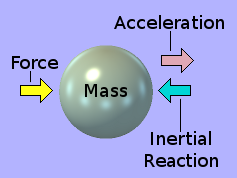When an accelerating force is applied to a sphere, the sphere applies an equal and opposite reaction against the applied force. This is known as an inert­ial reaction. Why does inertial reaction exist? From where does it come? Why does the applied force not simply induce the sphere to accelerate at an infinite rate? Conventional science answers that it is be­cause the substance of the sphere has mass and that the inertial reaction is a result of mass's in­her­ent reluctance to be perturbed.

This notion obviously comes from our everyday experiences of objects here on the surface of the Earth. From a universal perspective, however, this argument is rather nebulous. Mass is simply the constant of proportionality that relates an observed amount of force with an observed amount of acceleration for a particular object such as the green sphere. It sheds no light on the observation that the sphere ap­pears to be somehow connected to the rest of the universe, albeit in a sense which is one differential with respect to time removed from solid anchorage.

To me, a more cogent idea is that the sphere is immersed in — or is part of — some kind of hyper-fluid whose viscosity impedes the sphere's acceleration. I call it a hyper-fluid because, unlike a conventional fluid, it does not impede, in any way, the passage through it of an object travelling at constant velocity. In fact, for an object travelling at constant velocity, it isn't even there. It doesn't exist. It only manifests its existence when an applied force attempts to accelerate an object through it. The amount of impedance it offers is directly proportional to the object's acceleration through it. This hyper-fluid is what I have called the æther.

There is a very important difference, which must be noted, between a normal phys­ical fluid and the æther.

If the green sphere were moving through water, the resistance the water exerted would vary non-linearly with the sphere's velocity through it. This is because water can flow easily around the sphere at low velocities. However, as the velocity incr­eases, the water cannot pass round the sphere fast enough. This results in a pres­sure zone in front of the sphere, which increases in volume as the sphere's velocity increases.

If the green sphere is accelerating through the æther, this acceleration is strictly proportional to the force applied. This suggests that the æther does not or cannot "flow" around the sphere. The æther does not therefore act upon the surface of the sphere as water does. Instead, it appears to apply its inertial reaction at every point within the substance of the sphere. Furthermore, there is no detectable lag be­tween the application of the force and the equal and opposite inertial reaction. Every point within the substance of the sphere would thus appear to have a direct and immed­iate connection to the æther.

But which æther? Is inertial reaction a result of an object being accelerated relative to its own spherically-symmetrical temporal influx? Or is it a result of its interaction with the spherically-asymmetrical temporal influxes of the other other objects in the universe?

In the first case, for the universe to react to the object's acceleration through the object's own temporal influx, information about the object's acceleration would need to be pas­sed radially outwards from the object to the rest of the universe backwards along its in-flowing æther. Since the æther is flowing convergently into the object at the speed of light, this is clearly impossible. The information could not get out for the same reason that light cannot get out of a black hole.

A corollary is that the æthereal influx into a sink-hole must be universally constrained to remain always spherically symmetrical. Thus, accelerating the sink-hole will not perturb this symmetry.

However, the asymmetrical æther flow into other objects isn't flowing at light-speed — at least not in a transverse direction — relative to the object concerned. Conse­quently, the second case, of an object inscribing transversely onto another object's passing æthereal flow, is entirely feasible.The object has inertia — a reluctance to having its velocity changed. The inertial reaction exhibited by the object is the reaction from the passing æther of a dest­in­ation sink-hole to being disturbed [etched upon] by the lateral component of the object's motion. The lateral component of the object's motion is the object's motion along its path times the sine of the angle between its path and the direction of the passing æther. The æther, when thus perturbed, does not spring back to its original smooth state. It is malleable like metallic lead and thereby preserves the perturb­ation or etching all the way to its destination sink-hole.

Each sink-hole, of the object being accelerated, etches a transverse inscription onto the passing æther of one destination sink-hole. The sink holes of the object will thus write onto the æthereal inflows of destination sink-holes in every direction in space from the location of the accelerating object. The 'radiation' profile of the accelerat­ing object will thus have the form of the closed torus shown above, with the strong­est 'radiation' in the plane through the object that is perpendicular to its direction of acceleration.I surmise that the etching, which the object inscribes upon the passing æther of a dest­ination sink-hole, will have the nature of a transverse flux density curl [∇×ρ] in the form of a very small spherical cap within the spherical surface of the destination sink-hole's converging æthereal flow at which the accelerating object is located. The inscribing component of the object's acceleration can have any one direction within the 'plane' of the spherical cap. Thus the flux density curl inscribed by the accelerating object within the æthereal inflow of the destination sink-hole can be polarised in any one of this infin­ity of possible directions within this 'plane'.

As the spherical cap of æther, upon which the event has been written, progresses towards the observer, its area diminishes as follows.

Thickness of spherical cap: δr = cδt [deemed to be constant] = c [which is ∴ a constant] = ωr² [i]..... = (ωδr)r² = dV/dr × dr/dt [ii]..... = (2ωcδr)r

Thus, the rate of change of volume of the spherical cap diminishes linearly as the spherical cap proceeds towards the observer.

As the spherical cap of æther, upon which the event has been written, progresses towards the observer, the flux-density of the æther within it increases as follows.

Density of the æther: ρ Declare a new constant: Q = k/V = k/{(ωδr)r²} = k/(ωδr) [iii]..... = Q/r² = Q × (−2/r³) [iv]..... = −2Q/r³

Thus, while the flux-density of the æther within the spherical cap itself increases inversely as the square of its distance from the observer, its rate of change dimin­ishes as the inverse cube of the distance.

Further derivatives of the rate of change of æthereal flux-density with radius are:

 [v]..... 6Qr4 −24Qr5 120Q r6 −720Qr7 5040Qr8 and so on...

It is thus very clear that the rate of change of æthereal flux-density with distance is extremely non-linear. How relevant this is, to the mechanism whereby nature writes the etchings on the æther, I don't know.

The Complete Mechanism

I postulate that the complete mechanism that generates the inertial reaction when an object is accelerated by a directed external force is as shown below:The 7 stages of the cause-and-effect sequence as enumerated in the diagram are as follows:

1. A perturbing force is applied to an object,
2. which causes the object to accelerate.
3. This generates a perturbing influence upon the æther,
4. which causes it to undergo a change of state.
5. The æther resists this change of state
6. by returning an æthereal reaction to the object.
7. This causes the object to return a reactive force.

The energy expended by the Finger of God has thus been transferred to the æther, which subsequently conveys this changed state to its destination.

This overall mechanism gives rise to what I see as some relevant observations:

1. An individual object in free space has only a passive role in that, when acted upon by a directed external force, it cannot 'store' energy but only transduce it. This is because so-called 'kinetic' energy isn't real: it is only a perceived energy, which is different relative to every other body in the universe.

2. The state of the object is exactly the same after it has been forcibly acceler­ated as it was before it was forcibly accelerated; that is, it is at rest travel­ling along its world-line. It may be moving at a zero or greater constant velo­city or even accelerating non-linearly with respect to other objects.

3. A self-contained object in free space is thus not inherently able to undergo a change of state. It is not therefore a machine: only a passive transducer.

4. The æther, on the other hand, is caused to undergo a change of state as a result of the object being accelerated across the path of its flux. The æther may thus be said to be a machine. The transduced action of the force app­lied to the object impels it into a higher state, into which it apparently lat­ches. This transduced action is analogous to an input message.

5. In that the æther has a ground state and at least one elevated state, it may be viewed as a finite-state machine. The elevated state may comprise more than one sub-state as does an atom. Or it may simply have one higher state with a continuously-variable energy level.

6. There are several candidate means by which the accelerating object could transfer the energy expended by the original perturbing force onto the æther. Perhaps it causes a split or polarisation of some kind of the 'fabric' of the æther or a transverse 'curl' within its flux density. It could even induce some kind of complex-dynamical oscillatory effect, as in the energy states of an atom.

7. The æthereal machine also has an output, which is transduced into the force of inertial reaction exhibited by the object against the original perturbing force. In this sense, the æthereal machine returns a kind of acknowledge­ment message.

In this process, I surmise that each accelerating sink-hole within the accelerating object changes the state of the æthereal flux pertaining to only one destination sink-hole. Thus the whole object changes the states of the æthereal influxes of as many destination sink-holes within the rest of the universe as there are sink-holes within the material of the object. This one-to-one relationship can explain simply the notion of how a 'photon' can transfer energy point-to-point while collectively radiating in all directions and also what mainstream science refers to as 'particle entanglement': a one-to-one linkage between the accelerating sink-hole in the object and the destination sink-hole.

Is The Force-Wave Process Reversible?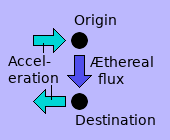What happens when æthereal flux in an altered state — the spherical cap of æther affected by an accelerating sink-hole — arrives at the destination sink-hole? My guess is that the æther gives up its stored energy, thus falling back to its ground state. This released energy accelerates the destin­ation sink-hole equally but in the opposite direction to that of the original sink-hole. The universe thereby is returned to its original state of energy balance, while having conveyed in­formation from the origin to the destination.

So yes, the process is reversible. But is the transfer of energy via the æther in this way detectable?

When an object such as the green ball is accelerated, all sink-holes within it raise any æther crossing its path of acceleration to a higher state. Notwithstanding, the destination sink-holes whose æther they affect need not — and most probably are not — part of one integral object. They're in separate objects in separate locations in separate directions within the universe.

The integrated set of sink-holes within one object is receiving the large composite inertial reaction from the æthereal fluxes of many destination sink-holes, However, since these destination sink-holes are distributed among many objects of any and all sizes, each will only receive a minuscule fraction of the energy expended by the perturbing force that accelerated the original object. This is most likely so small as to be below the threshold of detection by any practical means. In other words, the energy expended by the original force is extremely distributed.

Applying a force within the range of human experience to an every-day object like the green ball will only accelerate it relatively slowly to a humanly perceivable speed. Since the energy delivered to the æther is proportional to the square of the average velocity, it won't be all that great. So the energy received by any one destination sink-hole will be negligible. Detection will be impossible.

But suppose we accelerate a single sink-hole to near light-speed within the order of a microsecond or so. The square of the average velocity will then be enormous, resulting in a huge transfer of energy to the æther of a single destination sink-hole. But how can we do this?

The Notion of Charge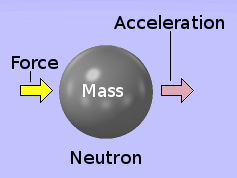A neutron may be perceived as an electrically-neutral subatomic object of a particular mass. As such, although vastly smaller, it could replace the green sphere in the foregoing discourse on force, mass and acceleration. According to my theory, a 3-dimensional vortex of neutral æther flows sym­metrically into the neutron. When it is acted on by a directed external force, the neutron, like the green sphere, exhibits an inertial reaction.

What is perceived as the mass m of the neutron is therefore the coefficient of inertial reaction k of the æther times the number of æthereal sink holes n within the neutron (m = k × n). Thus k is a property of the æther: not the neutron.

As part of an atomic nucleus, the neutron is indefinitely stable. On its own, how­ever, it decays fairly quickly, having a half-life of only 10 minutes 18 seconds. It de­cays into a proton and an electron.

The decay process is also said to liberate a proposed particle called an electron-anti-neutrino in order to balance the books re­garding the conservation of energy and momentum. During the decay process, the electron and electron-anti-neutrino re­main entwined (for about 3×10−25 second) as what scient­ists call a W boson.Notwithstanding, the imbalance in the conservation of energy and momentum is based on the notion of mass being a property of an object, and hence constant. But if, as I propose, mass be really a multiple of k [the coefficient of inertial reaction] and therefore a property of the æther, there is no absolute mandate that it should remain constant, especially in close-quarters interaction at the scale of fundamental particles. Discontinuities and singularities are anathema to nature. And it generally avoids these through close-quarters non-linearities. Thus, if the factor k were al­lowed to vary in this way, the electron-anti would be unnecessary.

The proposed electron-anti is electrically neutral like its parent neutron, al­though vastly smaller. The proton and the electron, however, both possess an addi­tional attribute. This is called charge. The proton has a positive charge. The electron has a negative charge. The terms positive and negative are simply conventions used to distinguish one from the other. They merely state that one is the opposite [or perhaps more appropriately, the complement] of the other. For instance, posit­ive and negative could just as well have been termed pro and con; right and left; up and down; male and female.

NOTE: the Standard Model of physics has conjured up a whole host of so-called fundamental particles, the vast majority of which are very short-lived, which suggests that they are nothing other than transitory states between stable and metastable particles. I also visualise the metastable particles not as 'solid' objects but as hives of complex-dynamical activity, which can spontaneously transmute into more stable forms.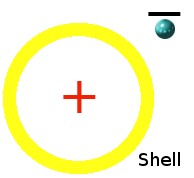So the proton shell containing the single positive charge [positron], would appear to be a mechanism that forces the segregation of electrical charge. It is a closed surface that enforces the spherical asymmetry of charge by act­ively capturing and engulfing positive charge while reject­ing and excluding negative charge. Each positive charge is thus encapsulated within its own protective bubble of exclusive space, leaving all negative charge unprotected and naked in the outer void.

Of course this bubble of protected space may not be simply a shell. It may be a sphere composed of some kind of æthereal essence, which could have a homogen­eous density or diminish with increasing radius like a kind of 'atmosphere'.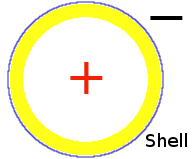The hydrogen atom has many discrete energy states that may or may not form a single multi-winged complex-dynamical attractor. Some of these states are stable while others are only meta-stable, each spontan­eously falling back to its next lower stable state. The very lowest state is a meta-stable state in which the electron becomes a kind of superficial atmosphere around the proton. Thus is formed what we call a neutron.

To a neighbouring proton in a composite atomic nucleus, the neutron would appear to have a negative charge in the form of an open-centred toroid. Thus, positioned between two protons, a neutron could bind or 'glue' them together by electrical force of attraction 'f' as follows:

f = q²/r² * (1 + 1/3² - 1/2²) = 0.861111111q²/r²,

where 'r' is the radius of the 'proton shell' described above.

Geometrically, the above formula is only approximate. However, further to this, the constant 'q' may not be constant. At macroscopic and indeed at microscopic scales, the so-called 'force of attraction' follows an inverse square law. Notwithstanding, at the nanoscopic scale of electrons and protons, 'q' could be a significantly non-linear function of distance, thereby making the binding force much stronger. Remember that nature often resorts to close-quarters non-linearities to circumvent what would otherwise give rise to awkward singularities. Of course, the function is continuous in reality. It is just that the non-linearity only kicks in strongly at close quarters.

I postulate that my proposed charge-segregator membrane, which encapsulates positive charges, works as follows. Its outer surface acts as a sink for the nega­tive component of the in-flowing æther. Its inner surface acts as a sink for the posi­tive component of the in-flowing æther.

What is perceived as the encapsulated positive charge within the closed surface of the membrane is the dynamic back-wash of the positive æther back-tracking its flow into the inner surface of the membrane. What is perceived as the excluded negative charge outside the closed surface of the membrane is the dynamic back-wash of the negative æther back-tracking its flow into the outer surface of the membrane.

In the case where electrons and protons are separate and unassociated, the inner and outer surface layers of the charge-segregator membrane could either separate or maintain a form of remote entanglement.

When protons and neutrons are in close proximity, as in the nucleus of an atom comprising multiple nuclides, the charge-segregation surfaces encapsulating the individual positive charges would distort from their normal spherical shape.

For the most part, this segregation of charge occurs only within the bounds of an atom. Above this the homogeneity in the distribution of charge makes matter in general electrically neutral. Notwithstanding there are specific circumstances on the macroscopic scale where charge can become segregated. A natural example is lightening. But charge is also segregated by artificial means like an electrostatic generator or radio dipole. Even so, this segregation takes place within the confines of material systems, like metallic crystals or agglomerations thereof.

Because it is encapsulated within its charge-segregation membrane, each positive charge pretty well stays put within the nucleus of its atom, which in turn is held in place within a molecule or a crystal lattice. It is only negative charge, which is unencapsulated, that can be moved independently. It can be moved between dis­crete energy levels within an atom or from a region of negative charge surplus to a region of negative charge deficit as with lightening. This movement generally in­volves negative charges [electrons] being accelerated.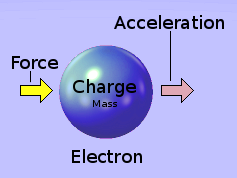Suppose, instead of accelerating a neutral object as discussed earlier, we were to accelerate an electron. An electron has a small neutral 'mass' associated with it. If a directed external force be applied to an electron, it accelerates at a rate determined by its minuscule mass. Of course, to accelerate an elect­ron, you need to apply a directed external force to it. But to do this, without resorting to the Finger of God, you need something other than a material object.

An Electron Under Forced Acceleration

The problem is, how do we apply a force to an electron? It is too small to push with a material device. The answer is that we do it with an electric field. But wait! If we make an analogy here between a so-called gravitational field and an electric field, the charge of an electron must be to the electric field what mass is to a gravita­tional field.

If mass falls within a gravitational field, it is suffering no force. It is simply going with the flow of the neutral æther. So if charge be analogous to mass, isn't an electron, falling within an electric field doing the same? Isn't it just going with the flow of the negative component of the æther? Superficially, it would seem so.

But an electric field is not analogous to a so-called gravitational field. An electron is an object which has what is conventionally perceived as a mass. When accelerating an electron, we are accelerating a 'mass' which is the same as any other object with a 'mass' — whether or not it possess an electric charge. The electric field, acting upon the electron's negative charge, is a separate and independent mechanism, which applies a real force to the electron's mass. The electron — as an object with 'mass' — is thereby accelerated through the velocity-fluid that I call the æther, from which, in return, it suffers an inertial reaction.

I have previously supposed that the æther comprise two complementary forms, which I call positive and negative. I shall now further suppose that an electron be a single sink-hole, which sinks only negative æther. Consequently, if we accelerate an electron, it can only affect the state of negative æther; namely the converging æthereal flux of another electron. Rather than the Finger of God applying a physical force to the electron, a strong electromotive force field accelerates the electron almost instantly to near light-speed.

The destination electron, the state of whose passing æther was altered by the ac­celerated electron, is thereby forcibly accelerated in the opposite direction. That is assuming that the destination electron be a 'free' electron within a vacuum or the atomic lattice of a conducting metal. If the destination electron be a bound electron, then it will be held in place within its parent atom.

A corollary to this is that all sink-holes must be either positive or nega­tive, each type having an æthereal influx of its respective polarity. Neutral matter — comprising equal numbers of positive and negative sink-holes — will therefore sink both types of æther equally. The much weaker resid­ual effect of neutral æther is due to an essential geometrico-dynamical asymmetry between the two types. Notwithstanding, the negative æther or temporal flux converging towards an electron is not of itself negatively charged in any electrical sense. In other words its temporal flux is not div­ergent [∇.ρ ≡ 0]. Likewise for positive æther.

Of course, what I have described here is an alternative abstract hypothesis for what mainstream science calls electromagnetic waves. These are not only readily detect­able but also readily producible in a controlled fashion. However, this hypothesis in­corporates a mechanism for the phenomenon of inertial reaction at both the macro­scopic and nanoscopic scales.

Natural Wave-Generators

There exist many mechanisms and processes in nature that etch event traces onto the passing æther. One such mechanism is an atom. The term passing æther refers to æther flowing radially-asymmetrically relative to the atom. Whatever the atom may etch upon æther flowing radially-symmetrically into itself can never be seen by the outside universe.In this context, an atom may be thought of as a complex-dynamical event-driven machine, as de­picted on the left, which can exist, at any given time, in any one of a finite number of discrete states. As such, it normally rests in its LOWest (or ground) state, which is ostensibly stable. On oc­c­asions it is pushed, by an external event , into a HIGHer (or excited) state . This higher state is only meta-stable. Consequently, after a seem­ingly random delay , the atom spontaneously falls back from its excited meta-stable state to its original stable ground state. In so doing, it inscribes a discrete modulation  upon the passing æther, which mainstream science refers to as a photon. Indeed, the original event , which triggered the whole sequence, could itself be a photon arriving on the atom's own radially symmetrical æthereal in-flow.An observer's in-flowing æther passes an atom as shown in the adjacent diagram. The area of the con­tour of equal density of this passing æther, upon which the atom can write, has the form of a spherical cap subtended by the solid angle ω. The atom spon­taneously falls from its excited state to its ground state. In so doing, it etches a trace, of varying amp­litude, up­on the passing æther. If the duration of the whole event be δt then the entire inscription of it is etched within a solid-angle shell of volume V = (A = ωr²) × (δr = cδt), where r is the distance be­tween the atom and the observer. Once written upon, this solid-angle-shell of æther continues its journey towards the observer at velocity c, maintaining its thickness, cδt, while its cross-sectional area, A, continually dim­inishes as it proceeds towards the observer.

I imagine the reading mechanism to be somewhat analogous to a magnetic read-head, which reads the signal from a magnetic tape passing by it. The only thing I can see as being relevant, therefore, is that, at the distance from the sink-hole at which the reading process takes place, the flux-density of the æther should be within the limits necessary to facilitate the reading process.

The æther, as I have conceived it in previous essays, flows into a sink-hole in the centre of each atom in the observer's retina. Notwithstanding, I think that the etch­ings within the æther must be read by the atom itself. I suspect that the reading process destroys or erases the etchings on the æther before it passes into the sink-hole.Whether the etchings, arriving on the æthereal in-flow, are read by the standing-wave structure of negative æther (electron cloud) surrounding the nucleus or by the concentric standing-wave struc­ture of positive æther (the nucleus itself) depends, I think, on the frequency — or more specifically, the rate of change of modulation depth (the rise rate or ramp gradient) — of the etched wave. I imagine that low-gradient waves are read within the peri­pheral region of sparse negative æther while high-gradient waves are read within the central region of higher-density positive æther, as illustrated by the adjacent diagram.

The reading process knocks the atom into a temporary meta-state from which it subsequently falls. The subsequent fall, at least for the slower rise rate waves of the visible spectrum, somehow triggers a signal in the observer's optical nerve, which conveys the signal to his brain, thereby giving him conscious sense of the event.

It would thus seem that the rules, by which the writing and reading processes take place, are as follows.

1. The signature of an event can only be written onto an æthereal flux that is flowing radially asymmetric­ally th­rough or across the writer.

2. The signature of an event cannot be written to the æthereal flux that is flowing radially symmetrically into the writer.

1. The signature of an event can only be read from the æthereal flux that is flowing radially symmetrically into the reader.

2. The signature of an event cannot be read from the æthereal flux that is flowing radially asymmetrically acro­ss or through the reader.

The writing and reading processes are themselves only the two end-points of the wave's journey as an inscription borne upon the observer's in-flowing æther. But what motivates the writing process? What powers it? It is the æther.My hypothesis is that the æther, of which the uni­verse is made, as represented by the large trans­lucent sphere below, continually drains radi­ally-symmetrically into sink-holes. Just as it is about to enter a sink-hole, the æther reaches a critical den­sity at which its positive and negative compon­ents, due to a slight spherical asymmetry between them, naturally separate into a standing wave structure of positive æther, which faces radially in­wards towards the sink-hole and a corresponding standing wave structure of negative æther, which is forced radially outwards to form a surrounding spherical shell. See illustration on the right.Borne upon each others æthereal in-flows, the sink holes accelerate towards each other. This causes them to congregate into clusters, as illust­rated on the left. The concentrically polarized standing-wave structure, surrounding each sink hole, repels others when in close proximity. This prohibits the sink-holes from merging. However, once the num­ber and density of congregated sink holes passes a certain threshold, the mutual repul­sion of their surrounding standing-wave back­wash­es of polar­ized æther becomes insufficient and the sink holes fuse together, thereby gaining larger more comp­lex æthereal backwash struct­ures.

Beyond this certain threshold of congregation density, the forced fusion of separate standing-wave structures into composite ones involves the separate accelerated compression of positive and negative æther. This means that separate regions of positive and negative æther suffer absolute accelerations. Depending on the forms and shapes of the standing-wave components involved, these accelerations can sometimes be radially symmetrical and other times not.

I propose that the crux of the writing mechanism be as follows. When a region of separate negative or positive æther is forcibly accelerated, it etches a mark upon the passing æthereal in-flow of a remote observer. Thus, the forced accelerations of the separate regions of positive and negative æther, which occur in the fusion process result in what is conventionally perceived as electromagnetic radiation.Of course, what I have just described, in terms of my own hypothesis, is what mainstream science would call gravity-powered atomic fusion. And this is what causes the stars to shine. Fusion is not the only mechanism in nature which forcibly accelerates regions of separated negative or positive æther thereby writing upon the æther inscriptions we think of as electromagnetic radia­tion. The thermal agitation of molecules and macro­scopic acceleration of regions of negative æther, such as lightening strikes, also write up on the æther. There are also artificial mechanisms.

Artificial Wave-GeneratorsOne such artificial mechanism is a radio dipole. The animation on the left shows a dipole powered by a transmitter [TX]. The red curve demonstrates how the electromotive force (or voltage) varies throughout the length of the dipole. Space above the horizontal black line of the dipole represents positive voltage and below it represents negative voltage. The voltage motivates an electric current to flow along the dipole. The green curve demon­strates how the amplitude of the current varies along the dipole. Note that the amplitude of the current follows 90° [π/2] behind the amplitude of the voltage throughout the repeating cycle.

Please note that the red and green curves in the above animation do not represent anything real occupying the space above and below the dipole. They merely represent graphically the electromotive force-field along the length of the dipole and the flow of electrons within its material.

The transmitter produces the varying electromotive force (voltage), which drives the varying current. In the conventional view, the current comprises a large herd of electrons moving along or through the conducting material of the dipole. These are being accelerated by the voltage, which is an electromotive force. Note that the electrons are not being pushed by a mechanical force against a reactionary electro­motive force. On the contrary, the electromotive force is the prime mover, which is countered by the reactive inertia of each electron's so-called 'mass'.

This is a cause and effect reversal of the situation with gravity. You can construct a mechanism to exert a mechanical force on a body to which the body's inertia will react. But you cannot construct a mechanism to generate a gravitational force field against which a body's inertia will react; especially not one that alternates sin­usoidally. I must conclude, therefore, that a field of electromotive force cannot be regarded as being analogous to a bipolar version of gravity. It is a phenomenon which is, conceptually, quite different. Unlike gravity, an electromotive force-field exerts a real mechanical force upon an electron.

I prefer to imagine the material of the electrical conductor, of which the dipole is made, as comprising a single standing-wave cloud of negative æther with zillions of small nuclei of positive standing wave structures nestling in regularly spaced niches of equilibrium. The alternating electromotive force field, produced by the trans­mitter, forcibly swills the outermost superficial layer of the negative æther cloud back and forth from one end of the dipole to the other.

The part of the red curve appearing above the dipole in the animation can be thought of as representing the amount of excess negative æther swilled towards its respective end of the dipole. The part of the red curve appearing below the dipole consequently represents the corresponding deficit of negative æther in the space left by the shifted negative æther.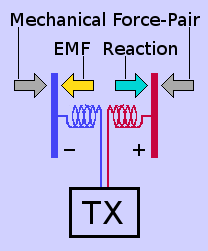The diagram on the right represents a dipole in terms of functional symbols. The electromotive force moves the superficial negative æther (conventionally known as electrons). It is a normal mechanical force. It therefore has a reaction, which acts against (gets its purchase from) the stationary material of the dipole, comprising the standing-wave structures of positive æther (atomic nuclei) surrounded by their respective standing-wave structures of negative æther (full elec­tron shells). The absence of the superficial negative æther at one end of the dipole leaves there an excess of positive æther within the nuclei. This results in a mechanical force-pair acting along the dipole as if to compress or shorten it.

In the above diagram, the EMF [electromotive force] is shown as a force that acts upon negative æther. It pushes electrons. This is the reverse of established conven­tion but it follows the sequence of cause and effect within the mechanics of my hypothesis. It would be interesting to know what would happen if the two halves of the dipole were not join­ed together rigidly but by a spring. The mechanical force-pair would then cause the two halves to be pushed towards each other and then spring back again for each cycle of the transmitter's driving frequency. [This is essentially the principle used in electrostatic loudspeakers, which were popular during the 1960s, 70s and 80s.] Would the resulting mechanical dissipation of the transmitter's power diminish the power of the æthereal radiation from the dipole?

All these forces are dominant only during the part of the electrical cycle at which the dipole is not etching anything upon the passing æther (or, in conventional terms, when it is not radiating). The æthereal etching effect, on the other hand, is dominant when the flow of superficial negative æther (electrical current) along the dipole reaches a maximum. And, as can be seen in the above animation, this occurs at the centre of the dipole.

Previously, I described the etchings as a form of stress inscribed into the passing æther. In retrospect, however, I now prefer to think of the æther more in terms of a 3-dimensional conveyor belt upon which a layer of smooth sand is resting. The accelerating electrons in the dipole disturb this passing smooth sand. The disturb­ance is preserved because there is no motive for the sand to re-smooth itself. It just stays as it was put, conveying the written mark onwards to the sink-hole into which it is 'flowing'.

Here it is necessary once again to remember that, in free space, things are one differential with respect to time removed from our perceptions formed within our terrestrial environment.

The presence of a constant current [electrons travelling at their terminal velocity within the conductor] merely maintains the æther in a constant altered state from when the current is not present. So the observer, into whose sink-holes the æther is flowing, would perceive nothing. It is only a current that is changing in intensity [accelerating electrons] that etches a feature into the passing æther. Thus, it is only a varying current [accelerating electrons] that can etch a detectable feature onto the passing æther.

Contrary to mainstream science, æthereal waves, in my hypothesis, are not electro­magnetic. They are not disembodied electric and magnetic force fields playing a game of throw and catch with kinetic and potential energy as they wing along their way at the speed of light. The electromotive force field, which accelerates the elect­rons within the conducting dipole, does not leave the vicinity of the dipole. Neither does its differential effect: its associated 'magnetic' field.

The emitted waves are instead æthereo-mechanical. Each is a mechanical etching within the passing æther [or temporal flux] converging at the speed of light towards a destination sink-hole. The destination sink-hole is a remote electron. The mechan­ical etching was itself created by the transverse acceleration, across the said temp­oral flux, of a sink-hole within one of the free electrons within the dipole conductor.

An electric field is bipolar. In a dipole conductor, it links centres of charge surplus and charge deficit. It is strongest along the shortest route bet­ween the two. This is along the length of the conductor. However, the electric field exists also outside the confines of the conductor, diminishing with radial distance from the centre-line of the conductor. Notwithstand­ing, in my hypothesis, the electric field does not then embark on an out­ward journey, disembodied from the centres of charge surplus and charge deficit from which it emanates.

The transmitter is a radio-frequency alternating electrical power source. It drives the dipole. The alternating current along the dipole comprises accelerating electr­ons. These write a regular alternating etching onto the passing æther of each obs­erver. Each observer can thereby detect [be aware of] the presence of the source of the signal. A regularly alternating etching of this kind cannot, however, convey any meaningful information apart from the fact that the source exists and is active.The adjacent animation represents, from the obs­erver's point of view, the situation where a source dipole is etching a regular cyclic signal onto pas­sing æther. The vertical red arrow is what we per­ceive as the electromotive force that drives the process. The horizontal blue arrow is the resulting accelerating flow of electrons that does the actual etching. The dipole lies along the horizontal axis of the animation. My vision of the etching process is that an accelerating electron creates a transverse density curl [∇×ρ] into the destination electron's passing æthereal flux, which causes it to invoke an inertial reaction against the EMF.

Mainstream science and engineering see the varying current driven by the altern­ating electric field [EMF] as creating what is perceived as an alternating magnetic field. Notwithstanding, what we perceive as separate electric and magnetic comp­onents are really the same thing seen from different points of view. The magnetic component is nothing more than a manifestation of the rate of change of the elec­tric component and vice versa.

Human beings have no specific senses for electric and magnetic force-fields. We only have instruments with which to transduce them into visual indications on, for example, a meter or an oscilloscope. The indications we get may be thought of as two mutually perpendicular "edge-on" views of the same phenomenon. What we are really looking at — from two disadvantageous angles — is a single phenomenon [a rate of energy transfer], depicted in the animation by the rotating green arrow.The cyclic etchings, arriving regularly upon the ob­server's in-flowing æther, do not carry any mean­ingful information. They are, nonetheless, capable of being wilfully modulated to convey symbolic int­elligence. In the above animation, the green rotat­ing vector remains at a constant ampli­tude, which is represented by the radius of the grey circle. However, if the amplitude of this vector were to be flipped back and forth between its full amplitude and half that amount in a predetermined way, as shown in the adjacent animation, it could be made to carry a sequence of digital bits, which could be made to represent meaning to the observer.

The sample of digital modulation above is shown for only the first quadrant (90 deg­rees) of the full (360 degree) cycle. The whole of the cycle can, of course, be mod­ulated to convey meaningful information.

Information conveyed in this way, between an originator and a recipient, is symbol­ic. It does not, within and of itself, contain any understandable meaning. It needs a context, which both the originator and the recipient already possess, in order for the recipient to know what the originator is saying. This context is called metadata, which is a vital key to unlocking the meaning from the received symbols.

The above artificial mechanism, for sending intelligent signals, still uses the same underlying natural mechanism to etch the æther as does the atom. It accelerates negative æther. The standing-wave structure of negative æther, which forms the outer shell around a sink-hole, is large enough to host the short fast accelerations of the orders required to etch visible light. The dipole, on the other hand, is able to host the longer slower accelerations, required to produce radio-frequency etchings, by using the composite standing-wave structure of negative æther composed of the entire lattice of atoms that forms the conducting material of the dipole.

An excited atom contains its own source of EMF. This drives the momentary acceler­ation of part of the negative æther within its outer standing-wave structure, causing it to etch a mark onto the non-radial æther flow of a single destination electron. In the dipole, on the other hand, the much larger quantity of free electrons in the conducting material is accelerated by the external EMF provided by the transmitter.

The Primality of Force

It is easy to see that the prime mover of the whole process, whereby a radio dipole etches a signal onto the passing æther, is plain mechanical force. Mechanical force is used to turn an electrical generator. This produces electricity, which powers the transmitter. The transmitter generates a radio-frequency EMF along the dipole. This accelerates electrons [negative sink-holes], which etch an alternating signal [flux density curl] into the passing æther. This cause-and-effect sequ­ence is summarized in the following diagram.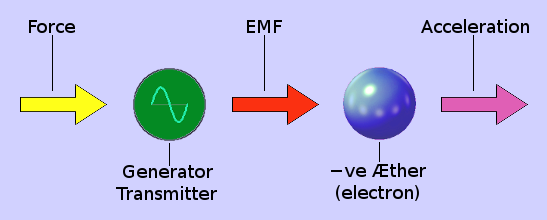The prime mover of the light produced by stars is also plain mechanical force. The radially-symmetrical flows of the æther into sink-holes causes them to accelerate towards each other. This causes them to congregate ever closer until they are on the point of merging.

The outer standing-wave structures of negative æther (electron shells), surrounding neighbouring sink-holes, resist each other's proximity by exerting real mutually rep­ulsive forces. But ever-increasing proximity causes the æthereal flux density to con­tinue to increase until the repulsive force of each sink-hole's outer shell of neg­ative æther (electrons) is no longer sufficient to keep them separate. Consequently, the outer shells of neighbouring sink-holes merge into a single standing-wave struc­ture of negative æther. Thus is formed what mainstream science would call a mole­cule.

The inner standing-wave structures of positive æther (nuclei), surrounding neigh­bouring sink-holes, resist each other's proximity by exerting vastly greater real mutually repulsive forces. But ever-increasing proximity causes this æthereal flux density to continue to increase until the repulsive force of each sink-hole's inner shell of positive æther (nucleus) is no longer sufficient to keep them separate. Con­sequently, the inner shells of neighbouring sink-holes merge into a single standing-wave structure of positive æther. Thus is formed what mainstream science would call a heavy nucleus.

In these processes, the work done by these two stages of repulsive force is liber­ated as æthereal waves.

Thus, in both these cases, it is ultimately mechanical force which — albeit indirectly — etches its marks upon the æthereal in-flow of every sink-hole in the universe. Hence, it is exclusively the wilful manipulation of pure mechanical force which en­ables us, as conscious beings, to communicate with each other.

Longitudinal Gravity Waves?

The inertial reaction of a body to an externally applied force is a very strong effect. But could there exist another infinitesimally smaller effect caused by the change in gravitational acceleration produced at a given target point by moving an object closer to it or further from it. Could this effect produce a detectable wave?

Although of vastly differing magnitudes, the "inertial reaction" created by accelerat­ing a body and the "gravity wave" created by displacing a body are both effects of perturbing æthereal flux. However, the vastly different magnitudes of these two effects is because each occurs within a very different context. Whereas the former concerns the relationship between one object and the rest of the universe, the latter concerns the much more localised relationship between two finite objects a finite distance apart.

I have described already in a previous essay the mechanism of mutual attraction between two finite bodies a finite distance apart in free space. The question now is, is it possible to generate a "gravity wave" of detectable magnitude using this much weaker phenomenon?

Consider two metal balls, joined by a thin bar to form a dumbbell, revolving around an axis through the centre of the bar. From any given point in space, the revolving balls appear to be exerting a gravitational acceleration 'a' that is modulated sinu­soidally to a magnitude 'δa' as the dumbbell rotates. The maximum effect is in the plane of rotation.In the above diagram, I have used a single constant 'Q = G × m', where 'G' is the universal gravitational constant [which is exceedingly small] and 'm' is the mass of each of the two steel balls. It is clear that this already very weak effect diminishes rapidly with distance according to the inverse-square law. But this is only the start. Since 'δr' is so vastly smaller than 'r', the amplitude 'δa' of the "gravity" wave, namely, the difference between 'a2' and 'a1' is an infinitesimal fraction of this al­ready infinitesimal effect.

Detecting such a weak effect would therefore be impractical with anything as crude as a similar steel ball. Furthermore, to get an effect of any detectable magnitude, the revolving balls would have to be something like a pair of black holes in a binary death dance.

For a wave to be created, energy must be expended. So does this phenomenon consume or transfer energy? The balls are not undergoing forced acceleration. So for them to continue revolving does not, of itself, require energy.

By revolving, the balls are simply changing the paths of the world-lines of all other objects in the universe on a cyclic basis. In so doing, the balls are not forcibly alter­ing the direction of anything. I cannot see therefore how the balls could be generat­ing anything that could be described as an energy-transferring wave. In any case, whatever change in æthereal flux density may occur within the vicinity of a distant object, that object could not possibly sense the change since no external directed force is involved.

A change in δa [see above diagram] will propagate, at the speed of light, across any object located at the target point [the vertical line at the right of the diagram]. If the object be a 1-metre diameter sphere, this will take about 3 nanoseconds. If it be a planet the size of the Earth, it will take about 43 milliseconds. As the change in δa passes across the diameter of the object, the path of the world-lines of successive slices of the object will change. Hence, infinitesimal tensile stresses will occur bet­ween successive slices as the change in δa passes across the object. Notwithstand­ing, this is yet one more degree of infinitesimalisation, rendering it of no practical significance other than theoretical completeness.

A Communicative Universe

The universe is, by definition, a single unified object. As such, all its parts — no matter how finely subdivided they may be — must be interconnected by some­thing. I have called that something the æther. In fact, I have gone as far as to say that all features within the universe must be merely complex knots and convolu­tions within the fabric of the æther. This means that all objects (or more correctly, sub-objects) in the universe must be connected. And if connected, they are related. Thus there must exist a tangible relationship between every pair of objects within the universe.As with two people, a relationship cannot exist between two objects without some form of communication taking place between them. Relationship cannot exist without communication. Consequently, any perturbation suffered by one object must, by virtue of its being a part of the universe, be communicated to all other objects within the universe. In other words, a perturbation suffered by one object within the universe, no matter how large or small that object may be, must necessarily and eventually be communicated to the whole of the uni­verse. Thus, if an object be perturbed by a force, exerted by the Finger of God, then that event must eventually affect [be communicated to] the whole universe.

So the universe communicates within itself: between its different parts. When one object within the universe is perturbed by a directed external force, this perturb­ation will eventually affect all other objects within it.

© 03Aug2015, 24Jun-15Jul2016, 27Nov2021 Robert John Morton | PREV | NEXT